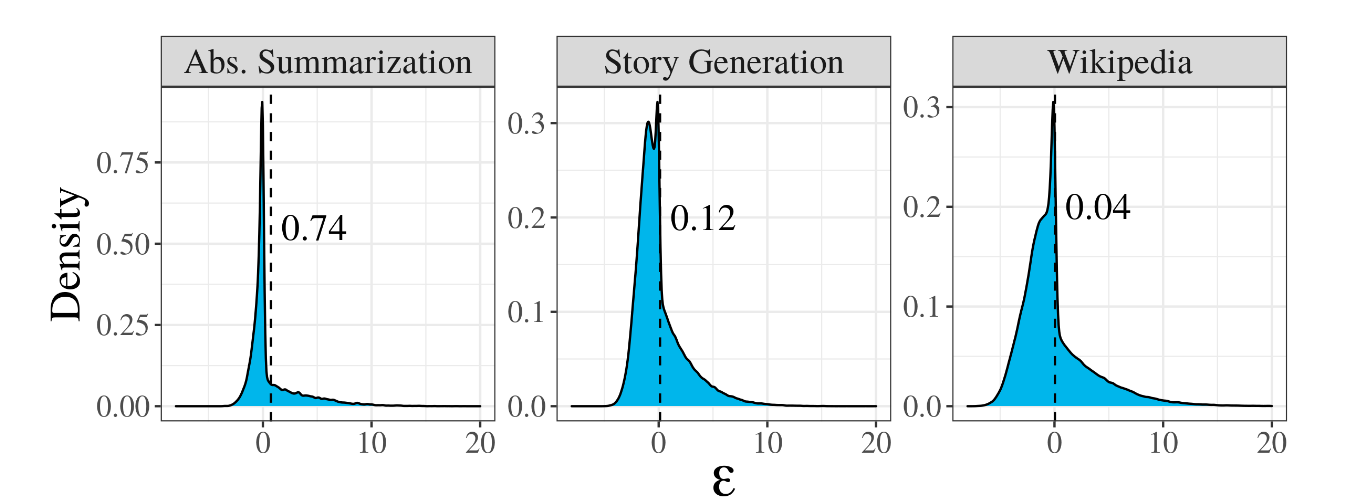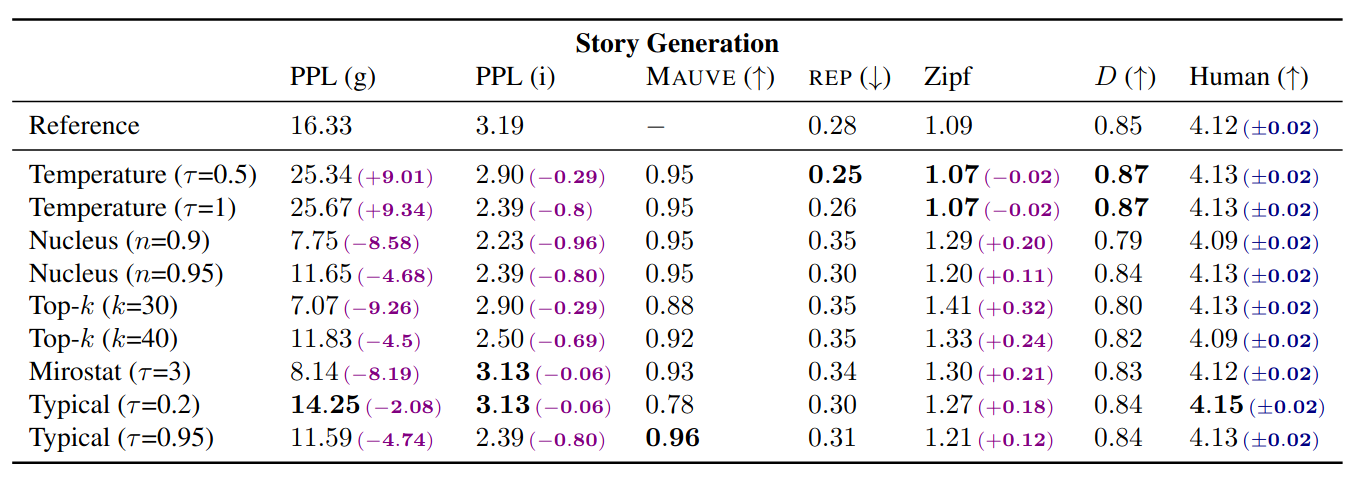# Latest Natural Language Generation Research Introduces ‘Typical Sampling,’ A New Decoding Method that is Principled, Effective, and can be Implemented Efficiently

Natural language models can model a text corpus C by learning the probability distribution p over all natural language strings Y∈C, with Y={y_1,y_2,y_3…,y_t}. For each word y_t, the model learns to output a probability distribution q over candidate words y∈V, the model’s vocabulary, choosing the words which minimize the log-likelihood of q(Y), which is the product of the probability of each word being in the sentence given prior context, q(y_t|Y<t).

Despite the learned distribution being optimal, in the sense that it minimizes the KL divergence between q and p, the process by which we should sample q to produce new natural language strings is unclear.

Suppose that, for every y_t we always picked the mode, that is, the candidate y which maximizes q. This is a reasonable strategy, and mode-seeking methods such as beam search have proven successful in text generation. Still, there’s an underlying problem: the sentence we just generated is, by definition, the most predictable, information-theoretically unsurprising sentence possible, which is not good if we go by the assumption that the goal of communication is to exchange information. This lack of information could also account for the repetitive loops found in AI text generation: language models often assign an increasingly higher probability to the repeated sub-strings of text, with each sub-string conveying less and less information after every occurrence.

In stochastic decoding, y_t is sampled randomly from q: despite solving the issue of repeated sub-strings, this often leads to incoherent text -one very unlikely word choice is will throw the entire context of the sentence out of the window.

A solution to this is top-k sampling, where y_t is chosen from the top-k most likely word candidates: top-k sampling often outperforms other methods in terms of qualitative metrics despite each string not being highly probable.

To account for this phenomenon, and in the scope of generating more human-like text, Clara Meister, Tiago Pimentel, Gian Wiher and Ryan Cotterell propose an alternative sampling method: “typical” sampling.

Typicality is a term that comes from information theory: a message is typical if its average per-symbol information content is close to the entropy rate of its source.

Human-generated text tends to be typical in that the information carried by a word in a sentence is close to the expected information the word should convey.

This claim can be tested empirically using language models: since we know the probability of a candidate word appearing at y_t, q(y | y<t), the information carried by the word, I(y_t), is its entropy, -log2(q(y | y<t)). By adding all the entropies of all candidate words for y_t, weighted by their probability, we can predict the expected information carried by y_t, E[I(y_t)] = −∑ q(y | y<t) * log2 q(y | y<t): |E[I(y_t)] – I(y_t)|<ε in human-generated text.So, to produce human-like text, we should choose a word whose information content is close to its expected information context.

For example, suppose we had three candidate words for y_t, with probabilities [0.2,0.5,0.3].

The expected information of y_t is E[I(y_t)] = -0.2*log2(0.2) -0.5*log2(0.5) -0.3*log2(0.3) = 1.48 bits. The information content of each candidate word is [-log2(0.2),-log2(0.5),-log2(0.3)] =

[2.3, 1.0, 1.73]: the word which accounts for most of the expected information content is the third one, which is our most likely candidate.

In the spirit of top-k sampling, the authors sample between words within a given threshold τ from E[I(y_t)] instead of picking a single word.

In experiments on two language generation tasks (abstract summarization and story generation), the authors find that typical sampling leads to a text of comparable or better quality than other stochastic decoding strategies according to human ratings. Further, when compared to these different decoding strategies, several quantitative properties of typically sampled text more closely align with those of human-generated text.As for why human-generated text is “typical”, the constant rate of information found in human-generated text might be a result of the attempt at maximizing the use of a communication channel: If a word conveys more information than the channel allows, there is a risk for miscommunication; simultaneously, if a word conveys very little information, then this channel is being used inefficiently.

It follows that there must be a “total” amount of information that the sender of a message wants the reader to receive: in order to produce typical sentences, this quantity must be planned ahead of time in order to evenly distribute it across words. To me, this seems to suggest that human text, whether it’s produced to ask for a favor, to prove a point or to elicit an emotion in the reader, is inherently goal-directed: what if instead of trying to make generated text mimic human text, we had AIs use communication as a means to an end, sampling whichever sequence of words maximized their utility function? Would the resulting text be “typical”? Perhaps in a future paper.

```This Article is written as a research summary article by Marktechpost Staff based on the research paper 'Typical Decoding for Natural Language Generation'. All Credit For This Research Goes To Researchers on This Project. Check out the paper.